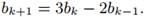# Consider a sequence of numbers b0, b1, b2,... such that b0 = 0, b1 = 1, and b2, b3,... are defined by the recurrence Find the value of bk.

Question
1 views

Consider a sequence of numbers b0, b1, b2,... such that b0 = 0, b1 = 1, and b2, b3,... are defined by the recurrenceFind the value of bk.

### This question hasn't been answered yet.